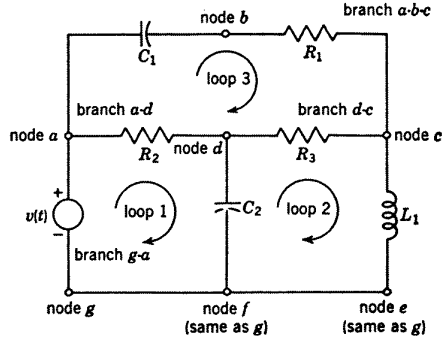### Some Important Key Points

Some Important Key Points:

• The junction formed by two or more elements being connected together is a node.

• A branch is an element in series such that identically the same current flows in them.

• A network or circuit is formed by interconnection of a number of branches or by coupling a number of separate parts together. Hence, a network is more complex than a circuit than a circuit as the network contains more elements as compared to circuit.

• A loop or mesh is a closed contour drawn on the schematic around one or more window panes.

• Any two nodes in a network are considered to be node pairs.

• Parts of a network not directly connected by wires but magnetically coupled are called separate parts of the network.

• A closed circuit is called a loop.

• A smallest part of the loop which cannot be further subdivided is called mesh

Note: In any network or circuit analysis the important factors are number of independent loos and number of independent node pairs.Here, in the above example, there are five nodes, seven elements and one part then number of independent loops(L) are

L = E - Nt + S
Where , E - No of elements

Nt - No of Nodes
S - No of parts